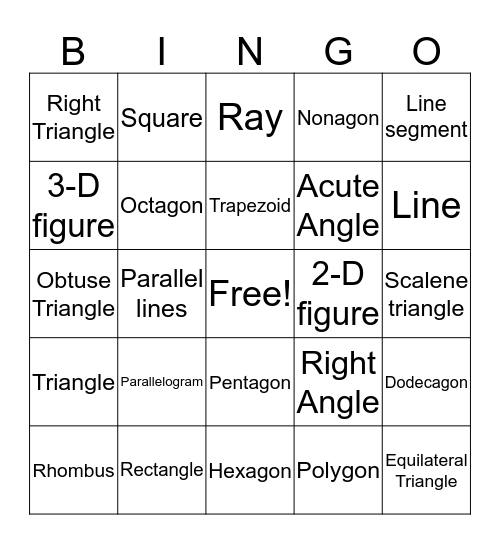# Geometry BingoThis bingo card has a free space and 24 words: Parallelogram, Rectangle, Trapezoid, Rhombus, Line segment, Right Triangle, 3-D figure, Square, 2-D figure, Pentagon, Acute Angle, Obtuse Triangle, Right Angle, Dodecagon, Octagon, Polygon, Scalene triangle, Ray, Line, Parallel lines, Equilateral Triangle, Triangle, Nonagon and Hexagon.

## Play Online